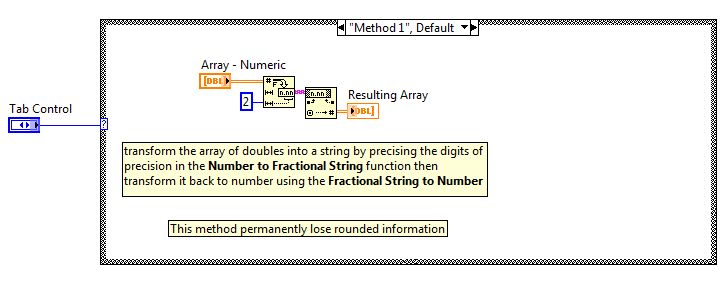# Example Code

## Code and Documents

### Attachment

Overview

This VI presents 3 methods to set the number of digits of precision in a DBL number.

Description

The following information can be found in this KnowledgeBase article.

Method 1:

1. Wire the double-precision value into a Number to Fractional String VI. The bottom input to this VI is the precision, or number of digits after the decimal place. The output of this VI should be a string containing the number with the correct significant digits.
2. To convert this back into a double-precision number, simply use a Frac/Exp String to Number VI. The output will be the number with the correct precision. This method also works for arrays of double-precision numbers.

Method 2:

1. Wire the double-precision value to a numeric indicator.
2. Right-click on the indicator and go to either Properties or Format Precision, depending on the data type (array or single number).
3. Under Format   and Precision, make sure the Precision Type is set to Significant Digits and then change the Digits numeric to the desired number.
4. Click OK to save this configuration.  Now you can create a local variable off of the indicator and use the value out from it.

Method 3:

1. Multiply the double-precision number by a multiple of 10 to move the decimal place.
2. Use the Round to Nearest VI to round the number off to an integer.
3. Then divide by the same multiple of 10 to move the decimal point back.

Requirements

LabVIEW 2012 (or compatible)

Steps to Implement or Execute Code

1. Download the attached VI Set Significant Bits of Double Precision Data_LV2012_NI Verified

2. Choose a method by selecting the tab

3. Run the VI

Note that some of these methods may permanently lose information so you can't restore the other digits of precision after conversion as for method 1 and 2.

Block DiagramFront Panel**This document has been updated to meet the current required format for the NI Code Exchange. **

Regards,

Sara Lewandroski
Applications Engineer | National Instruments
http://www.ni.com/support

Example code from the Example Code Exchange in the NI Community is licensed with the MIT license.Member
on

Is there a bug inside LabVIEW?

Just change under Format and Precision the Digits numeric to e.g. 26 and you will see that if you convert a

INT 942 value to a Double value you will get

941.999999999999999 ...

If I use e.g. a .NET language I get by a Double division of 94200/100=942.0

With LabView I get 941.999999999999999

DerIngMember
on

Which one of the three methods is most efficient to use for a large amount of data and wouldn't take much computing?

I am feeling Method #2 is.

Any idea?Member
on

Does it work to format data to output file (tdms)?